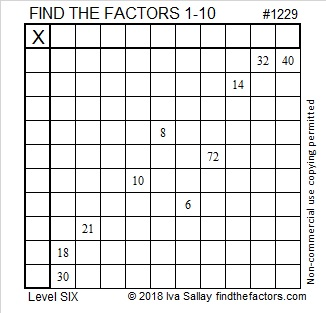# 1229 and Level 6

The only common factors permitted for 32 and 40 in this puzzle are 4 and 8, but which one will work for this puzzle? Likewise, you must decide if 3 or 6 is the right common factor for 18 and 30. Don’t guess which factor to use. Study the other clues and let logic guide your decisions until the unique solution is found. Have fun with this one!Print the puzzles or type the solution in this excel file: 10-factors-1221-1231

This is my 1229th post, so I will tell you a little bit about the number 1229:

• 1229 is a prime number.
• Prime factorization: 1229 is prime.
• The exponent of prime number 1229 is 1. Adding 1 to that exponent we get (1 + 1) = 2. Therefore 1229 has exactly 2 factors.
• Factors of 1229: 1, 1229
• Factor pairs: 1229 = 1 × 1229
• 1229 has no square factors that allow its square root to be simplified. √1229 ≈ 35.057

How do we know that 1229 is a prime number? If 1229 were not a prime number, then it would be divisible by at least one prime number less than or equal to √1229 ≈ 35.1. Since 1229 cannot be divided evenly by 2, 3, 5, 7, 11, 13, 17, 19, 23, 29 or 31, we know that 1229 is a prime number.1229 is the sum of three consecutive prime numbers:
401 + 409 + 419 = 1229

1229 is the sum of two square numbers:
35² + 2²  = 1229

1229 is the hypotenuse of a primitive Pythagorean triple:
140-1221-1229 calculated from 2(35)(2), 35² – 2², 35² + 2²

Here’s another way we know that 1229 is a prime number: Since its last two digits divided by 4 leave a remainder of 1, and 35² + 2² = 1229 with 35 and 2 having no common prime factors, 1229 will be prime unless it is divisible by a prime number Pythagorean triple hypotenuse less than or equal to √1229 ≈ 35.1. Since 1229 is not divisible by 5, 13, 17, or 29, we know that 1229 is a prime number.

This site uses Akismet to reduce spam. Learn how your comment data is processed.